Conge 精进

# Reinforcement Learning 第九周课程笔记

2015-10-18

This week

• Watch Generalization
• The readings are Gordon (1995) and Baird (1995).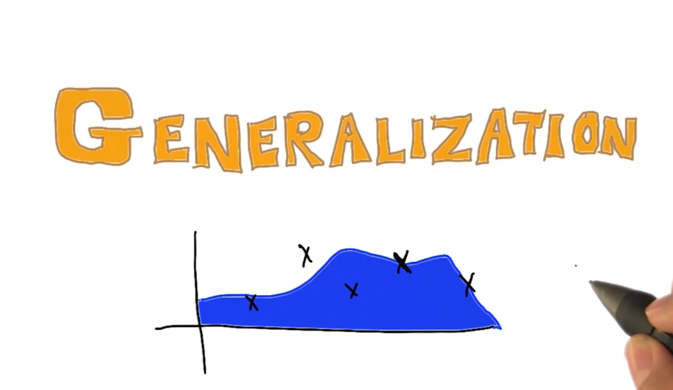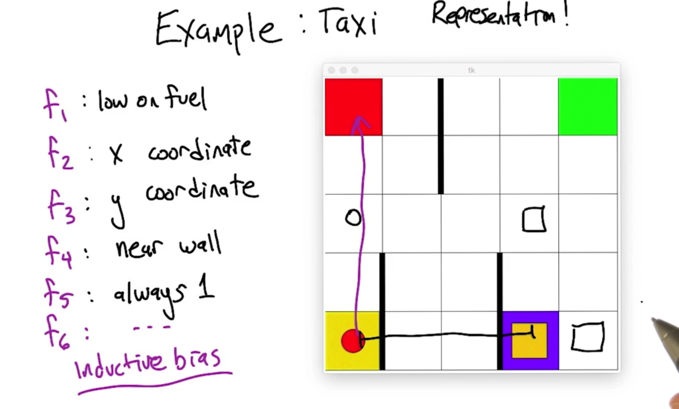• When there are a lot of states, we can use features to represent states (e.g. grouping states using some common features or using some base states to represent other stats)
• Inductive bias: refer to the way algorithm prefer one solution against another.
• representation can stay unchanged even when states changed(?)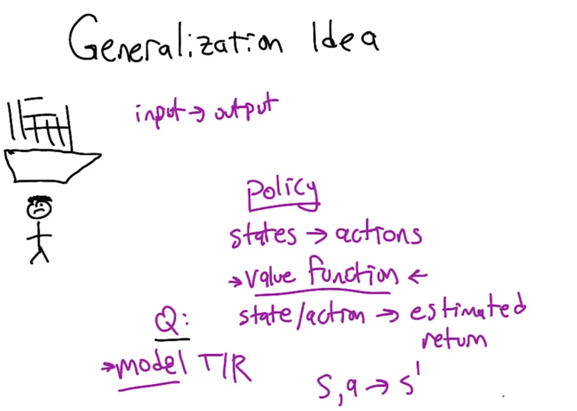• In RL, the goal is to learn some input-output mapping.
• e.g. Policy maps state -> actions
• value function maps state/actions to expected return
• Model maps T/R (transition and reward)
• Generalization can happen on all these levels
• Most researchers are focused on value function approximation.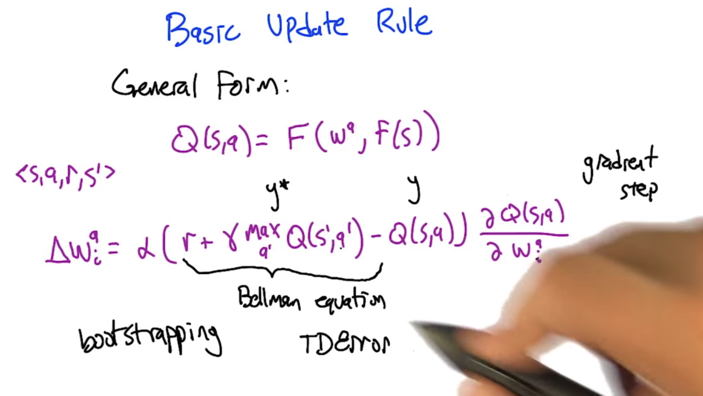• Q is represented by function approximator F(wa, f(s)). wa is some kind of weights, f(s) takes a state s and generate a series of features.
• experience tuple <s,a,r,s’> comes in, and do an update.
• TD error= the difference between predicted value (reward + discounted value of next state) and value of current state. TD error tells us the direction to move the parameters w. (the prediction is high, low or just right).
• α is learning rate.

## Linear Value Function Approximation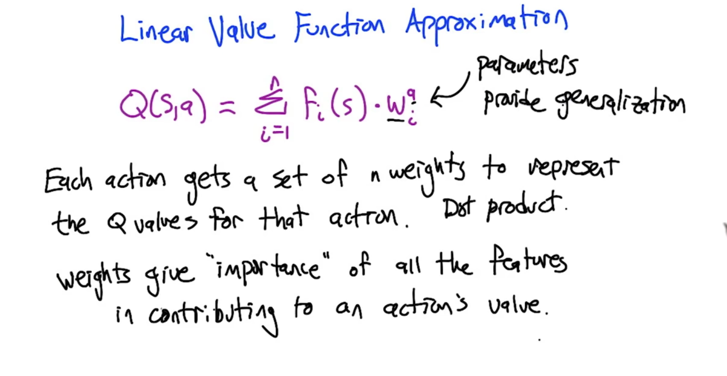• Q value for an action is represented by features and a set of weights
• wai are weights of features fi(s), which represent importance of features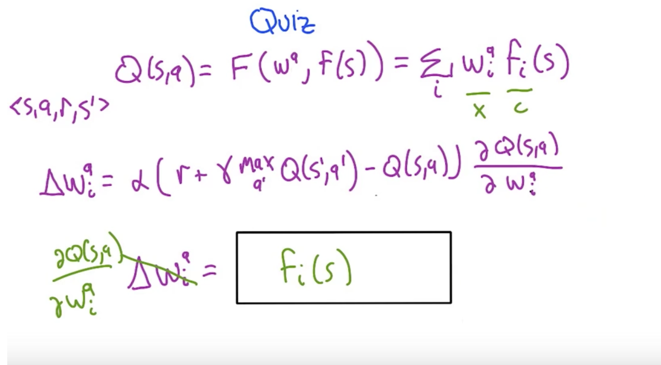## Success and fail stories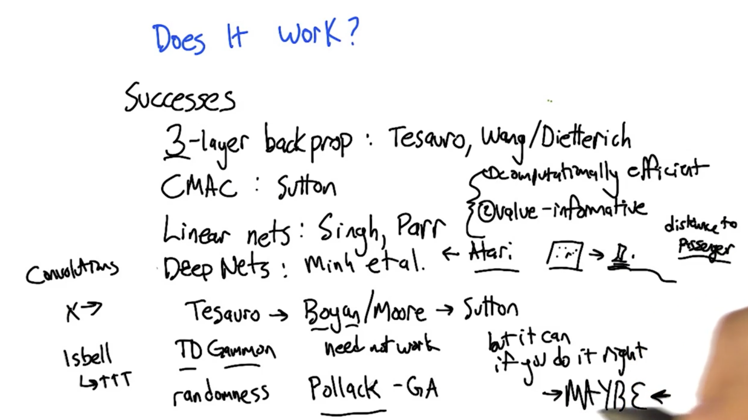• 3-layer back prop: decision making in Jeopardy
• CMAC(wikipedia) :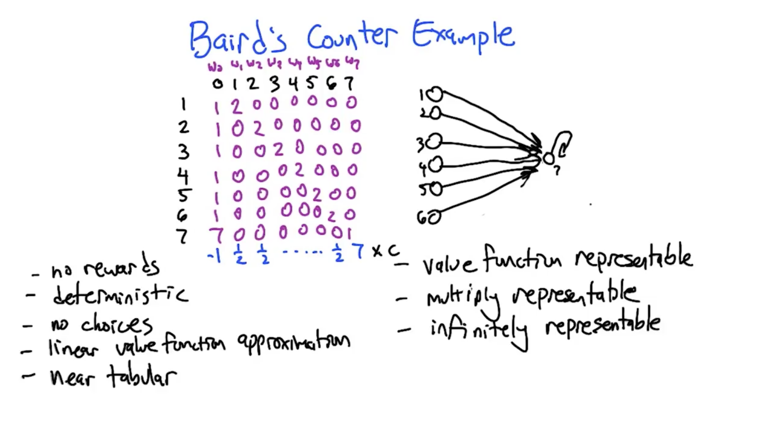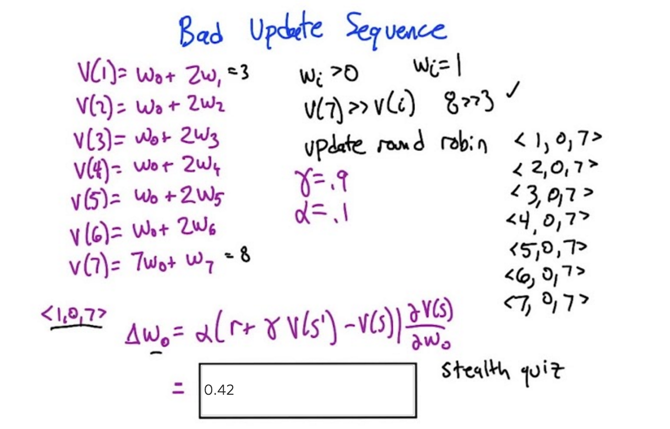• V(s’) = V(7) =8; V(s) = V(1) =3, derivative is w =1; so Δw0 = 0.1( 0 + 0.9 * 8 -3)*1 = 0.42;
• Δw0 is 0.42, and Δwi, i = [0, 6] are all positive, so weight will increase with each update
•  Δw7 is negative when we update <7,0,7>, but w7 will still increase because it’s original value is » Δw7
• So, even the algorithm is doing the right thing, the updates will never converge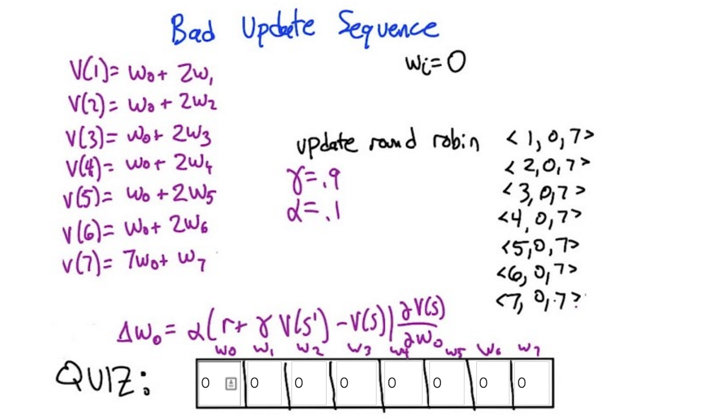• in this case, the temporal differences are all zeroes because wi is 0, which means no updates (changes) are going to happen. Sticky
• If at any circumstance, when all values are zero, it could not escape the circumstance unless reward (r) is non-zero. If reward is also zero, we can call the circumstance the solution of the MDP.
• this holds when MDP is deterministic.
• the lesson: Shared weights can “not converge”!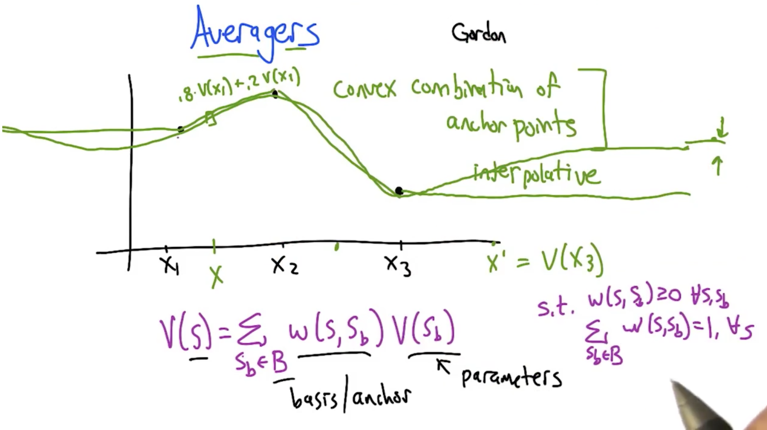• Problem: given a certain points of value, estimate the function of line. How to estimates the points in-between?
• Naturally, weighted average of neighbours and get convex combinations of anchor points
• for points that are far from anchor points, are good estimate will be the average of the anchor points.
• more anchor points lead to less error in MDP estimation.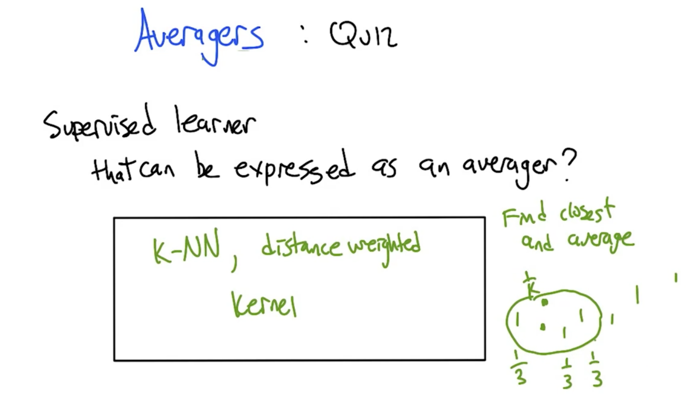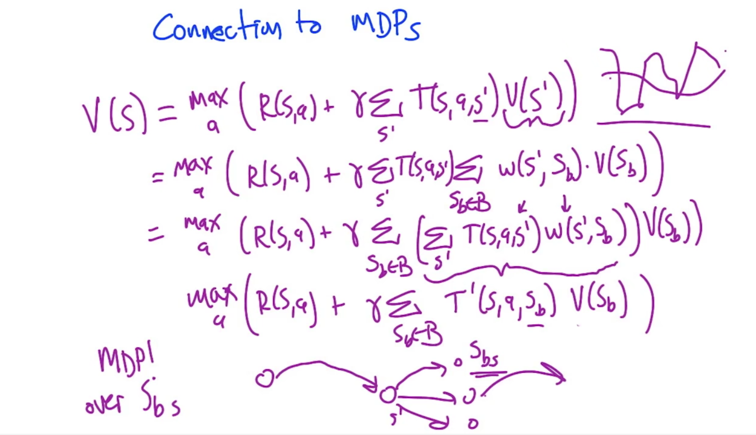• Using base states, we can respresent the original MDP with MDP over Sbs

## What have we learned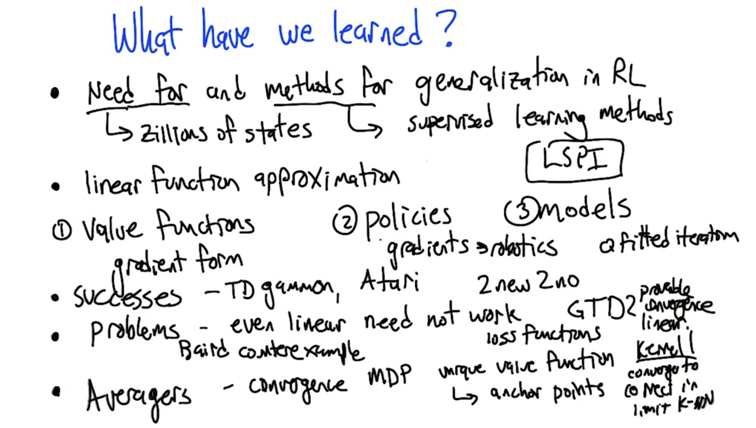• Need for generalization: some problem have Zillions of states; Method: applying supervised learning method .
• Methods: Linear function approximation.
• 1) value functions 2) policies; 3) models;
• Success: TD gamma, Atari, Fitted Q-iteration (but still have need not work cases)
• Problem cases: even linear need not work (baird counter problem)
• Averagers: can be viewed as MDP itself. Use anchor points to represent the whole MDP.
• LSPI: Least Squared policy iteration
``````2015-10-13 初稿 upto quiz 1
2015-10-17 完成
2015-12-04 reviewed and revisted
``````